##### Quantum Physics For Dummies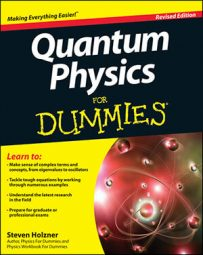If you’ve read through the last few sections, you’re now armed with all this new technology: Hermitian operators and commutators. How can you put it to work? You can come up with the Heisenberg uncertainty relation starting virtually from scratch.

Here’s a calculation that takes you from a few basic definitions to the Heisenberg uncertainty relation. This kind of calculation shows how much easier it is to use the basis-less bra and ket notation than the full matrix version of state vectors. This isn’t the kind of calculation that you’ll need to do in class, but follow it through — knowing how to use kets, bras, commutators, and Hermitian operators is vital in the coming chapters.

The uncertainty in a measurement of the Hermitian operator named A is formally given by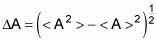That is,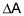is equal to the square root of the expectation value of A2 minus the squared expectation value of A. If you’ve taken any math classes that dealt with statistics, this formula may be familiar to you. Similarly, the uncertainty in a measurement using Hermitian operator B is

Now consider the operators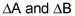(not the uncertaintiesanymore), and assume that applyingas operators gives you measurement values like this: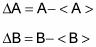Like any operator, usingcan result in new kets: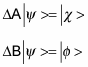Here’s the key: The Schwarz inequality gives you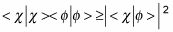So you can see that the inequality sign,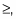which plays a big part in the Heisenberg uncertainty relation, has already crept into the calculation.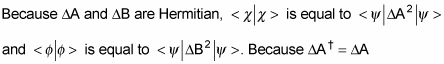(the definition of a Hermitian operator), you can see that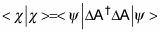This means that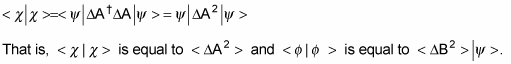So you can rewrite the Schwarz inequality like this: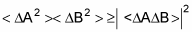Okay, where has this gotten you? It’s time to be clever. Note that you can write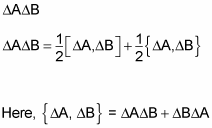is the anticommutator of the operators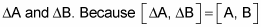(the constants and subtract out), you can rewrite this equation: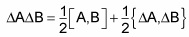Here’s where the math gets intense. Take a look at what you know so far:

The commutator of two Hermitian operators, [A, B], is anti-Hermitian.
The expectation value of an anti-Hermitian is purely imaginary.
The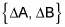is Hermitian.
The expectation value of a Hermitian is real.

All this means that you can view the expectation value of the equation as the sum of real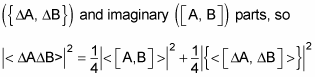And because the second term on the right is positive or zero, you can say that the following is true: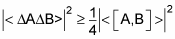Whew! But now compare this inequality to the relationship from the earlier use of the Schwarz inequality: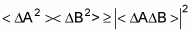Combining the two equations gives you this: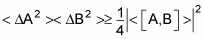This has the look of the Heisenberg uncertainty relation, except for the pesky expectation value brackets, < >, and the fact thatappear squared here. You want to reproduce the Heisenberg uncertainty relation here, which looks like this: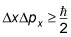Okay, so how do you get the left side of the equation from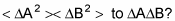Because an earlier equation tells you that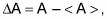you know the following: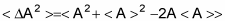Taking the expectation value of the last term in this equation, you get this result: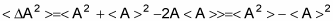Square the earlier equation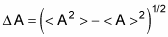to get the following: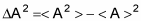And comparing that equation to the before it, you conclude that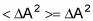Cool. That result means that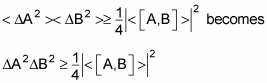This inequality at last means that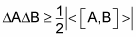Well, well, well. So the product of two uncertainties is greater than or equal to one-half the absolute value of the commutator of their respective operators? Wow. Is that the Heisenberg uncertainty relation? Well, take a look. In quantum mechanics, the momentum operator looks like this: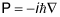And the operator for the momentum in the x direction is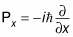So what’s the commutator of the X operator (which just returns the x position of a particle) and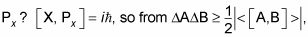you get this next inequality(remember,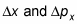here are the uncertainties in x and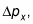not the operators):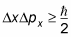Hot dog! That is the Heisenberg uncertainty relation. (Notice that by deriving it from scratch, however, you haven’t actually constrained the physical world through the use of abstract mathematics — you’ve merely proved, using a few basic assumptions, that you can’t measure the physical world with perfect accuracy.)